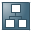Show TOC##The Interest Calculation Process### Process Flow

The interest calculation process is as follows:

...

1.      The program identifies the items on which interest is to be calculated according to the rules defined in the interest indicator, and any additional specifications you make when executing the program. The rules you enter determine the items and transaction for which interest can be calculated, such as:

Cleared or open items only

All clearing transactions or only those with a payment (thereby excluding uncleared credit memos and payments on account).

Credit and debit items or only debit items. If interest is to be calculated on credit items (for example, credit memos and payments on account), it is calculated in the same way as for debit items.

2.      The program determines the days for which interest is to be calculated, taking into account the calendar type.

There are basically two factors involved in interest calculation: the lower and upper limit of the calculation period you specify for the interest calculation run, and the time period between the net due date and the date of the payment document (or the clearing date for credit memos determined per item). The program selects those items in which the period between the net due date and clearing date falls within the lower and upper limit of the calculation period. If the lower limit of the calculation period is suppressed, the system uses the value from the Last key date field in the master record as the lower limit.

You can specify whether interest should be calculated as of the date of the last interest calculation. That is, only those items still open as of the date of the last run will be included in the new run. Cleared items are only included if the clearing date is later than the date of the last interest calculation.

You can specify tolerance days for the interest indicator. These are added to the due date for net payment, but are only relevant for selecting the items to be included in the interest calculation run. Interest is always calculated as of the due date for net payment without taking into account any tolerance days. You can also define transfer days for cleared items. This is a way of making allowance for when payments take longer than usual to transfer or when the relevant accounts are not cleared promptly. Transfer days are subtracted from the date of the payment document or from the clearing date.

3.      After determining the items on which interest is to be calculated, the program works out the amount in local currency. In doing so, it takes into account any interest rate changes within the calculation period, and if a minimum amount is specified for the interest indicator, it compares the calculated interest amount with the minimum amount. By specifying a minimum amount, you can prevent letters from being created and sent to vendors for insignificant interest amounts.

4.      Correspondence is created if the calculated interest exceeds the minimum amount. The form containing information for the correspondence is defined in the interest indicator. However, you can specify a different form in each interest calculation run.

### Result

The letters you send to your business partners about interest calculation contain the following information:

Text

Overview of line items

Interest rate overview

Interest overview

The system also creates an error list if the calculation report did not run correctly.

You can also request the following lists:

Log

Account overview